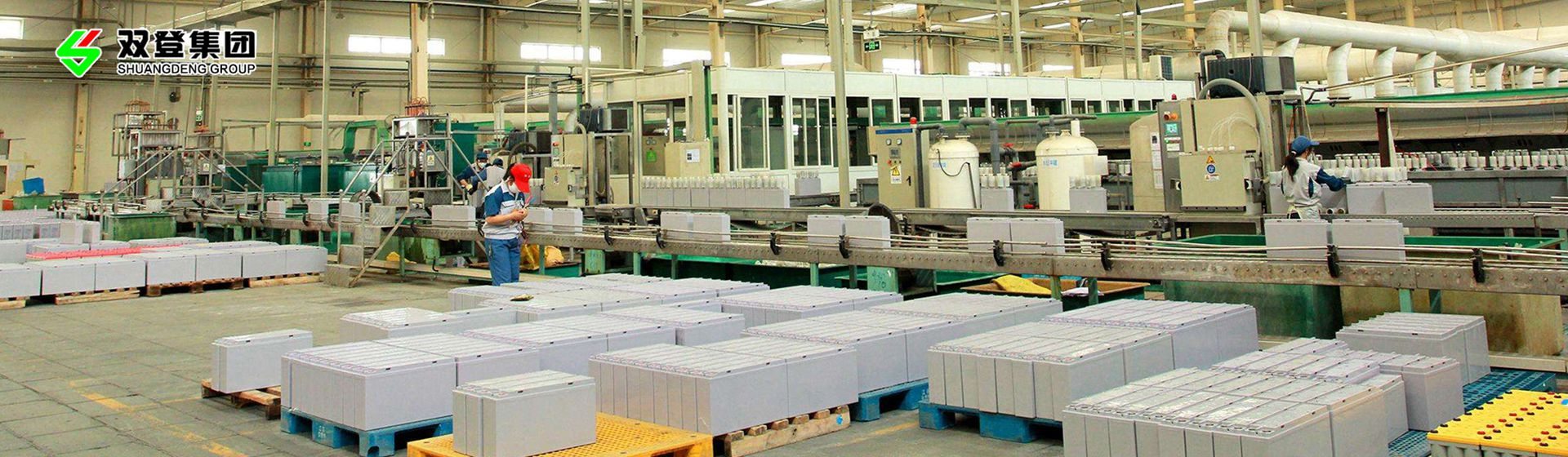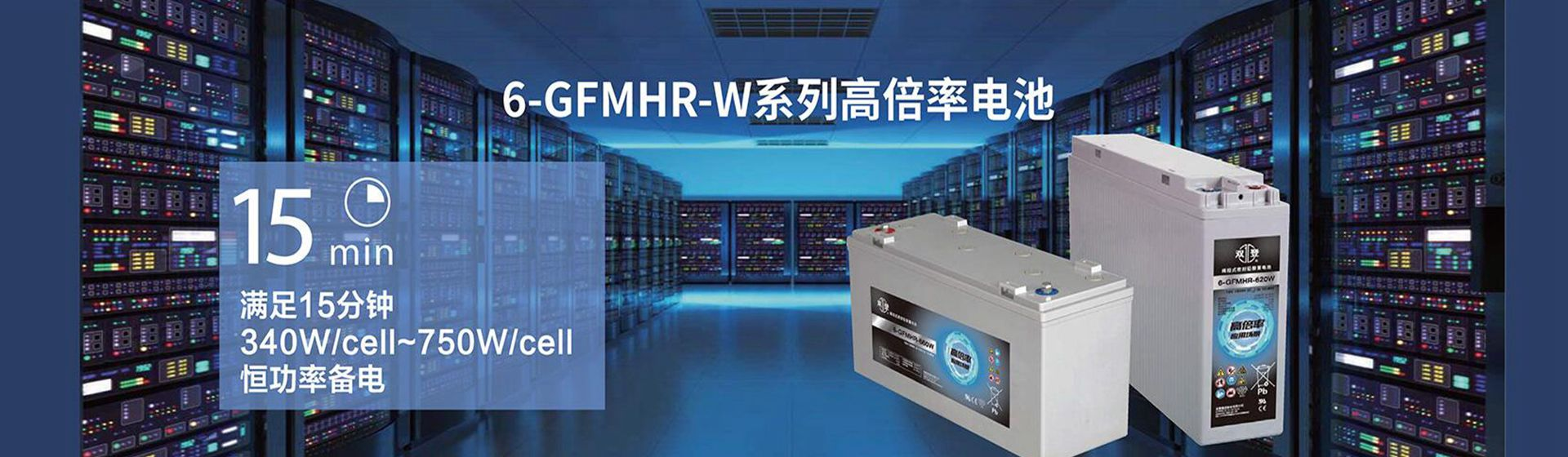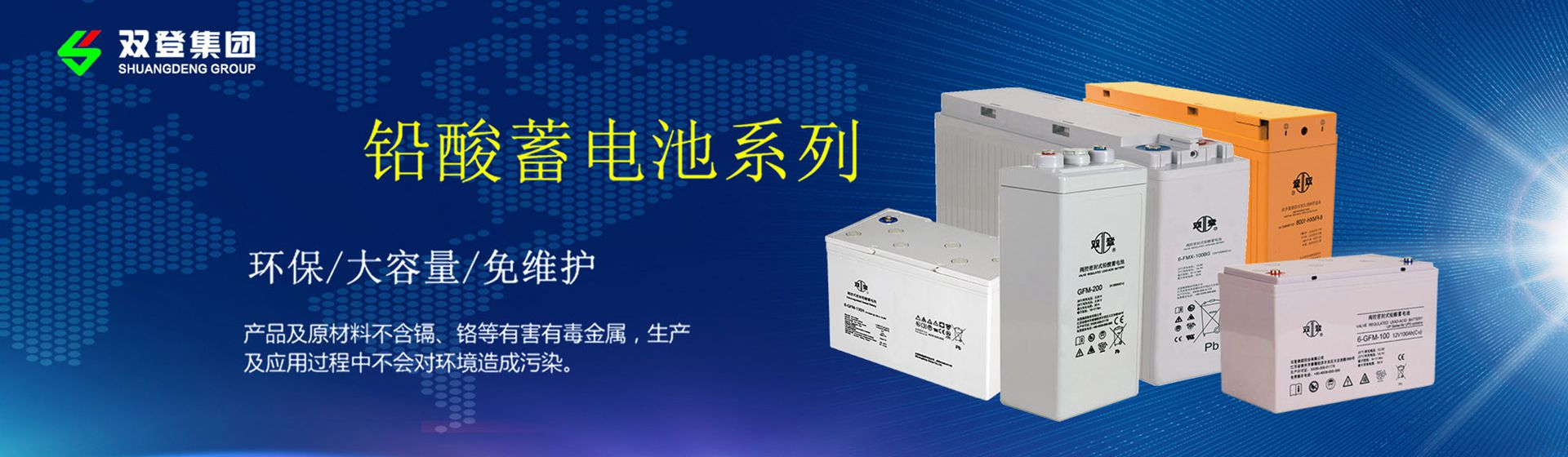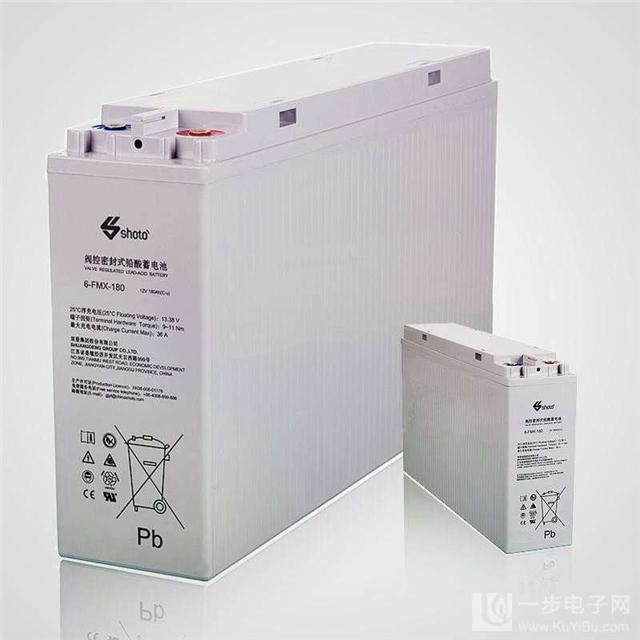- 产品展示 -
Product display

# 新旧蓄电池电池混用的弊端R1=3Ω（灯负载电阻）

I1=0.375A

I2=0.322A,说明混用电池小，此时落在旧电池内阻上的电压降为Vr2,Vr2=r2•I2=5×0.322=1.61V＞E2，它说明旧电池对电路的贡献小于它的消耗，这就是新旧电池串用灯，往往灯亮度更暗的原因。

I1=（r1+RL）+I2RL=E1

I2=(r2+RL)+I1RL=E2则：

4I1+3I2=1.53I1+8I2=1.4可得：

I1=0.339A  I2=0.048A

IL=I1+I2=0.387A

I=0.017A,即将有20mA的等效自放电电流，按电池容量为3A时计算，放电时间为T，T=176h=7天，即一周后新电池将放电殆尽。总之，新旧电池混用，不管串联还是并联，弊端很多，所以新旧电池不应混用。